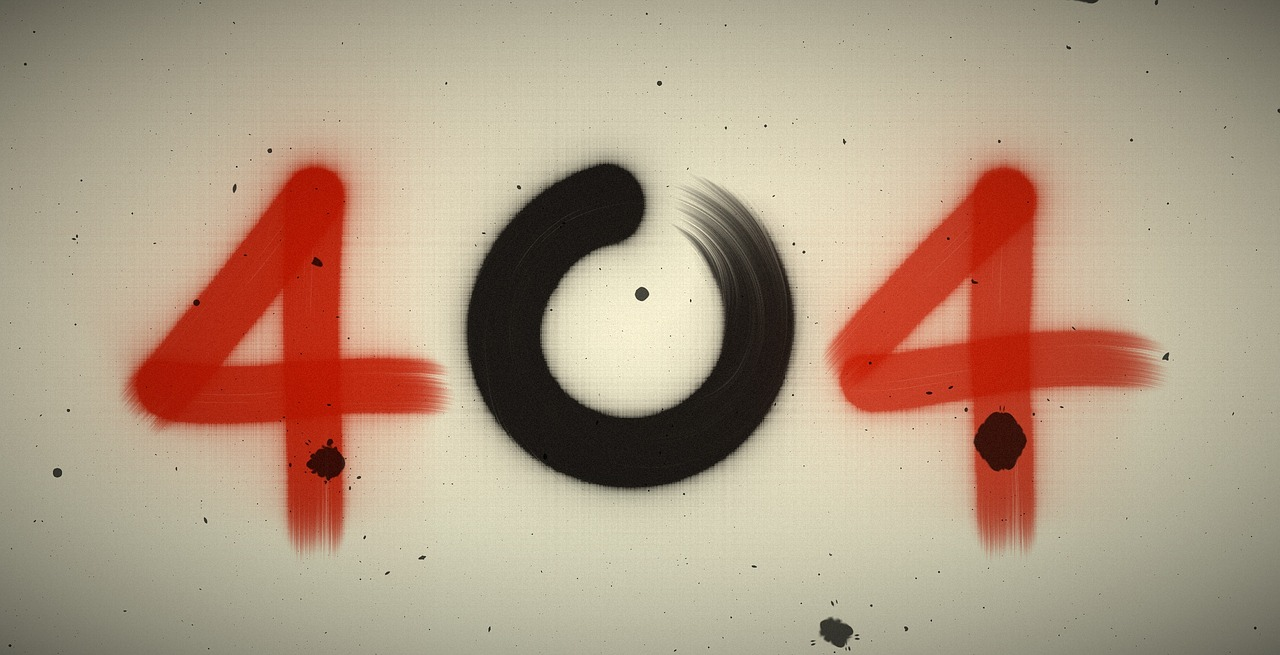## Post per Page

The idea that the universe is a hologram has been misinterpreted, perhaps partially due to the popularity of films such as The Matrix.

The laws of physics demand that we cannot see through a black hole's event horizon; it is fundamentally impenetrable. Even light cannot escape it.

But in a new study, a physicist from the University of Michigan has proposed a technique to do just that, and his findings could help solve one of science's most challenging problems.

The study, published in the journal PRX Quantum, even adds weight to the theory that the universe is a hologram.

Wait, the universe is a hologram?

The idea that the universe is a hologram has been misinterpreted as the theoretical physics equivalent of Plato's Cave, an ancient allegory about mankind's inability to truly perceive reality, perhaps partially due to the popularity of films such as The Matrix.

But the theory, formally known as Holographic Duality, is actually a mathematical model to reconcile one of science's greatest conundrums.

"In Einstein's General Relativity theory, there are no particles, there's just space-time. And in the Standard Model of particle physics, there's no gravity, there's just particles," explained Enrico Rinaldi, a research scientist at the University of Michigan.

"Connecting the two different theories is a longstanding issue in physics - something people have been trying to do since the last century," added Dr Rinaldi, one the paper's authors.

What is Holographic Duality?

Holographic Duality suggests that the theory of gravity and the theory of particles are actually mathematically equivalent. The problem is the theory of gravity requires three dimensions, while the particle theory only has two.

Black holes are a valuable object for the sake of reconciling these theories. They are so dense that they warp three-dimensional spacetime, but we observe them because of their mathematical connection to particles, effectively projected through two-dimensional space.

One of the mathematical tools used to represent particle theory are known as quantum matrix models. Solving these models would show that the maths which represent particle theory could equally represent gravity.

But solving them is a hard problem. The models are basically blocks of numbers that represent particles. They are solved by finding the best configuration of particles to represent the system's lowest energy state.

Quantum computers, such as IBM's, are not yet powerful enough to solve most matrix models

Quantum computers

To find the solution, the team used quantum circuits - doing the equivalent of shaking a bucket of sand to level out the top - and ran the system through a special neural network to find the wave function of the matrix with the lowest possible energy.

The problem is that most modern quantum circuits are not powerful enough to solve these models if they are too complicated, so Dr. Rinaldi and his team had to develop an exceptionally simple one to prove that their approach works.

As the technology develops they hope that researchers will be able to solve even more complicated matrix models.

"Because these matrices are one possible representation for a special type of black hole, if we know how the matrices are arranged and what their properties are, we can know, for example, what a black hole looks like on the inside," added Dr Rinaldi.

"What is on the event horizon for a black hole? Where does it come from? Answering these questions would be a step towards realising a quantum theory of gravity," he said.

He added that the results have provided an important benchmark for future work on quantum computing and machine learning algorithms that researchers can use to explore quantum gravity through the idea of holographic duality.

Story Written by Alex Martin